# Triangles-Important Questions

Class X Math
Hots For Triangles
1.  1. In the fig., P and Q are points on the sides AB and AC respectively of ��ABC such that AP = 3.5 cm, PB = 7 cm, AQ = 3 cm and QC = 6 cm. If PQ = 4.5 cm, find BC.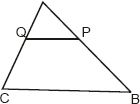2.  The lengths of the diagonals of a rhombus are 30 cm and 40 cm. Find the side of the rhombus.
3.  In the fig., PQ ‖ BC and AP: PB = 1 : 2. Find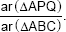4.  The perimeter of two similar triangles ABC and LMN are 60 cm and 48 cm respectively. If LM = 8 cm, then what is the length of AB?
5.  In ΔABC shown in figure, DE ‖ BC. If BC = 8 cm, DE = 6 cm and area of ΔADE = 45 cm2, what is the area of ΔABC?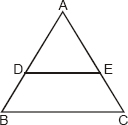6.  If the areas of two similar triangles are in ratio 25 : 64, write the ratio of their corresponding sides.
7.  If one diagonal of a trapezium divides the other diagonal in the ratio 1:3. Prove that one of the parallel sides is three times the other.
8.  In the given figure, ΔABC and ΔDEF are similar, BC = 3 cm, EF = 4 cm and area of ΔABC = 54 cm2. Determine the area of ΔDEF.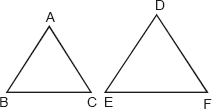9.  A right triangle has hypotenuse of length q cm and one side of length p cm. If (q � p) - 2, express the length of third side of the right triangle in terms of q.
10.  In the given figure, ABC is a triangle in which AB = AC, D and E are points on the sides AB and AC respectively, such that AD = AE. Show that the points B, C, E and D are concyclic.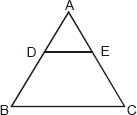11.  ABCD is a trapezium with AB ‖ DC in which diagonals AC and BD intersect at E and AAED ˜ ABEC. Prove that AD = BC.
12.  ABC is a triangle. PQ is a line segment intersecting AB in P and AC in Q such that PQ ‖ BC and divides ΔABC into two parts equal in area. Find BP/AB,
13.  ABC is a triangle in which AB = AC and D is any point in BC. Prove that : (AB)2 – (AD)2 = BD . CD.
14.  AD is the median of ΔABC, O is any point on AD. BO and CO produced meet AC and AB in E and F respectively. AD is produced to X such that OD = DX. Prove that AO : AX = AF : AB.
15.  In a triangle ABC, P divides the sides AB such that AP : PB = 1 : 2, Q is a point on AC such that PQ ‖ BC. Find the ratio of the areas of ΔAPQ and trapezium BPQC.